# Difference between revisions of "Flory-Huggins theory"

The Flory-Huggins theory (although chronologically speaking it should be known as the Huggins-Flory theory ) for solutions of polymers was developed by Maurice L. Huggins  and Paul J. Flory , following the work by Kurt H. Meyer . The description can be easily generalized to the case of polymer mixtures. The Flory-Huggins theory defines the volume of a polymer system as a lattice which is divided into microscopic subspaces (called sites) of the same volume. In the case of polymer solutions, the solvent molecules are assumed to occupy single sites, while a polymer chain of a given type,$i$, occupies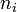$n_i$ sites. The repulsive forces in the system are modelled by requiring each lattice site to be occupied by only a single segment. Attractive interactions between non-bonded sites are included at the lattice neighbour level. Assuming random and ideal mixing, i.e. mixing volume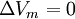$\Delta V_m = 0$, it is possible to obtain the well-known expression for the combinatorial entropy of mixing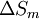$\Delta S_m$ per site of the Flory-Huggins theory for the general case of a mixture of two components, A (polymer or solvent) and polymer B (Eq. 10.1 of Ref. 3):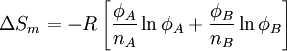$\Delta S_m = -R \left[ \frac{\phi_A}{n_A} \ln \phi_A + \frac{\phi_B}{n_B} \ln \phi_B \right]$

where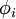$\phi_i$ is the volume fraction of the component$i$, and$R$ is the molar gas constant. Applying the concept of regular solutions and assuming all pair interactions in the framework of a mean-field theory yields for the enthalpy of mixing (Eq. 20 of Chapter XII in ):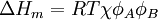$\Delta H_m = RT \chi \phi_A \phi_B$

where$T$ is the absolute temperature. According to the preceding equations, the Flory-Huggins equation can be expressed in terms of the Gibbs energy function of mixing for a binary system :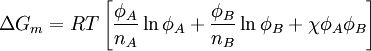$\Delta G_m = RT \left[ \frac{\phi_A}{n_A} \ln \phi_A + \frac{\phi_B}{n_B} \ln \phi_B + \chi \phi_A \phi_B\right]$

where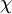$\chi$ is the dimensionless Flory-Huggins binary interaction parameter (similar to the van Laar heat of mixing ), which can be expressed as (Eq. 21 of Chapter XII):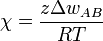$\chi = \frac{z\Delta w_{AB}}{RT}$

where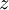$z$ is the coordination number and (Eq. 17 of Chapter XII)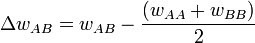$\Delta w_{AB} = w_{AB} - \frac{(w_{AA}+w_{BB})}{2}$

where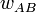$w_{AB}$ is the net energy associated with two neighbouring lattice sites of the different polymer segments for the same type or for the different types of polymer chains. Although the theory considers$\chi$ as a fixed parameter, experimental data reveal that actually$\chi$ depends on such quantities as temperature, concentration, pressure, molar mass, molar mass distribution. From a theoretical point of view it may also depend on model parameters as the coordination number of the lattice and segment length.

For polymers of high molecular weight (i.e.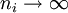$n_i \rightarrow \infty$) the entropic contribution is very small and the miscibility or immiscibility of the system mainly depends on the value of the enthalpy of mixing. In this case, miscibility can only be achieved when$\chi$ is negative. Assuming a$\chi$ temperature-dependent parameter, T vs.$\phi_i$ phase-separation diagrams can be constructed. For long polymers, miscibility can only be achieved when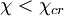$\chi < \chi_{cr}$. The$\chi$ parameter at the critical point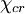$\chi_{cr}$ can be obtained from the definition of the critical point and the Flory-Huggins expression for the free-energy of mixing. The result is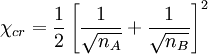$\chi_{cr} = \frac{1}{2} \left[ \frac{1}{\sqrt{n_A}} + \frac{1}{\sqrt{n_B}} \right]^2$

Therefore:

• Positive values of$\chi$ necessarily lead to immiscibility for polymer mixtures of high molecular weight.
• Polymer mixing always take place if the$\chi$ parameter is negative. Miscible polymer mixtures with negative$\chi$ exist due to specific interactions between given polymer segments. Miscibility or compatibility can be induced by several methods. For instance, introducing opposite charges in the different polymers or adding a copolymer containing A and B segments.
• For polymer solutions (whose sites have the volume of a solvent molecule,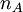$n_A$=1), the critical Flory-Huggins parameter is close to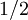$1/2$. The temperature corresponding to this value$\chi$=$1/2$ would be the critical temperature if the polymer is infinitely long and defines the theta temperature of the polymer-solvent system.
• For polymer mixtures,$\chi$ should be referred to the arbitrarily chosen microscopic volume defined as a site, e.g. 100 Angstroms. Usual values are close to zero 

The$\chi$ parameter is somewhat similar to a second virial coefficient expressing binary interactions between molecules and, therefore, it usually shows a linear dependence of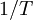$1/T$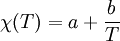$\chi(T) = a + \frac{b}{T}$

where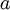$a$ and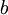$b$ are assumed to be constants, but can actually depend on density, concentration, molecular weight, etc. A usual interpretation is that$a$ represents an enthalpic quantity and$b$ an entropic contribution, although both$a$ and$b$ are actually empirical parameters. According to this description, the systems should show an upper critical temperature. Many polymer mixtures and solutions, however, show an increase of$\chi$ for increasing temperatures (negative entropic contribution) what implies the existence of a lower critical temperature.